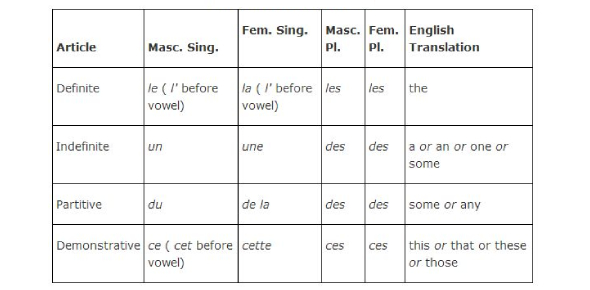A Quiz On French Articles!

8 Questions | Total Attempts: 4815SettingsWhat are French articles? French articles combine a noun to indicate the type of reference being made by a noun. Most of the time, articles specify the grammatical definiteness of the noun. Examples are the a and n. The definite article in French has three forms which correspond to the English article, “the. ” They are Le, La, and Les, and usage depends on the number and gender of the noun. You must take this amazing quiz and learn more about French articles.

• 1.
De la is definite. (True, False)
• A.

True

• B.

False

• 2.
La is feminine. (True, False)
• A.

True

• B.

False

• 3.
French articles are required on almost every noun. (True, False).
• A.

True

• B.

False

• 4.
Le, La, Les are equivalent to the English 'the'. (True, False)
• A.

True

• B.

False

• 5.
Each gender shares the same article. (True, False)
• A.

True

• B.

False

• 6.
Masculine Singular is le. (True, False)
• A.

True

• B.

False

• 7.
Femine Plural l'. (True, False)
• A.

True

• B.

False

• 8.
When the noun begins with a vowel or silent 'h', the le or la simply becomes l'. (True, False)
• A.

True

• B.

False

Related TopicsBack to top